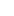# Tutor Hunt Questions

a) Indicate true or false to the following statements (no explanations needed):
i) Diversification is a sensible reason for mergers because it is cheaper for
corporations to diversify their business portfolios than for investors to diversify
their portfolios.
ii) Agency costs arise from the senior management’s inability to control
shareholders.

Suppose the total value of the firm is £150 million. The firm’s debt-equity ratio is 0.5, and the interest rate (before tax) on its debt is 4 percent, while its cost of equity is 12
percent. The effective corporate tax rate is 25 percent. By assuming that the Modigliani-Miller Theorem with corporate taxes holds, answer the following questions.
i) What is the value of equity of the firm?
ii) What is the after-tax WACC (weighted average cost of capital) of the firm?

A firm has the following capital structure: 100 million shares outstanding, trading at £1.5 per share, and £100 million of debt. The beta of the firm’s stock is 1.5. The firm’s cost of equity is 10 percent, and the yield on riskless bonds is 2.5 percent.There is no tax. Assuming that the firm can borrow at the risk free rate and that both
CAPM (Capital Asset Pricing Model) and the Modigliani-Miller theorem hold, answer the following questions.
i) What is the total value of the firm?
ii) What is the WACC of the firm?
iii) What should be the expected return on the market portfolio?
iv) Suppose the firm changes its capital structure so that its debt increases to £150 million, and the equity decreases by £50 million. What should be the firm’s cost of equity after the change?
3 years ago